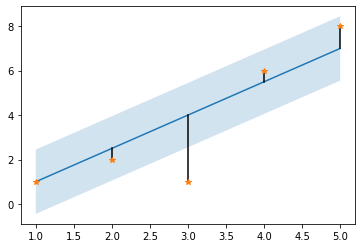Zindi Error Metric Series: What is Root Mean Square Error (RMSE)?
Getting started · 17 Feb 2022, 16:23 · 3 mins read ·
11

When working on a machine learning project, choosing the right error or evaluation metric is critical. This is a measure of how well your model performs at the task you built it for, and choosing the correct metric for the model is a critical task for any machine learning engineer or data scientist. Root Mean Squared Error or RMSE is a metric commonly used for regression problems, and is related to standard deviation.

For Zindi competitions, we choose the evaluation metric for each competition based on what we want the model to achieve. Understanding each metric and the type of model you use each for is one of the first steps towards mastery of machine learning techniques.

RMSE is a common error metric, as it penalises large errors, works in the same units as the target variable and it is quick to compute. However, it can be difficult to interpret. As RMSE is closely related to standard deviation, you need to keep in mind the context of the problem. If your target measure is a large number, your RMSE will be large; if your target measure is small, it will be a much smaller number.

For example: In our AirQo Ugandan Air Quality Forecast Challenge, RMSE values are ~30 because the target is air quality measured from 1-100ppm, so ~66% of values fall within 30 units of the true value. In our Hulkshare Recommendation Algorithm Challenge, RMSE ~0.001. This looks like a better answer, but the target is measured in the order of 10-3.

The Root Mean Square Error (RMSE) is often used in regression problems. You can visualise this error by the standard deviation of your predictions, as RMSE is usually used when the target follows a normal distribution.Ŷ are the predicted values

Y are the actual values

n is the number of observations

By squaring the difference between the actual and predicted values (the residuals), we ensure that all values are positive, thus avoiding them canceling out. The sum of all the residuals are summed and divided by the number of observations, taking the average.

In this image, if the blue straight line is the true values and the orange data points are predicted values, the residual is the distance between the predicted point and the corresponding point on the blue line.As you can see, outliers are penalised as they add a large residual value. When you are building your machine learning solution that will be evaluated using RMSE, think of an interesting way to take outliers into account.

Remember for RMSE: the lower your results, the better!

Here is a piece of code that can help you visualise what a straight-line RMSE would look like.

`import numpy as np`
`import matplotlib.pyplot as plt`

`# True values`
`x = np.linspace(1,5,5)           # x values`
`y = 1.5*x - 0.5                  # function that calculates the true y values`
`y_predicted = [1, 2, 1, 6, 8]    # predicted y_predicted values`

`# calculating the RMSE step by step`

`# step 1 - calucalte the residuals`
`residuals = (y-y_predicted)**2`

`# step 2 - sum the residuals`
`residuals_sum = residuals.sum()`

`#step 3 - divide by the number of values`
`mean_squared_error = residuals_sum/5`

`#step 4 - square root answer`
`RMSE_calculated = np.sqrt(mean_squared_error)`
`RMSE_calculated`

`# Using the Scikit library, we can calculate the same value.`

`from sklearn.metrics import mean_squared_error`
`RMSE_sklearn = mean_squared_error(y, y_predicted, squared=False)`
`RMSE_sklearn`

`# Viewing our true values (blue), predicited values (orange), residuals (black), 1st standard deviation/RMSE in pale blue`
`plt.plot(x,y)`
`plt.plot((x,x),(y, y_predicted),c='black')`
`plt.plot(x,y_predicted,'*')`
`plt.fill_between(x, y-RMSE_calculated, y+RMSE_calculated, alpha=0.2)`

With this knowledge, you should be well equipped to use RMSE for your next machine learning project. Why don’t you test out your new knowledge on one of our knowledge competitions that uses RMSE as its evaluation metric? We suggest the Urban Air Pollution Challenge.